#### Temporal differences

Rather than waiting until the end of each trial to compute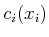, it is possible to update each state,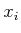, immediately after it is visited and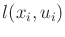is received from the simulator. This leads to a well-known method of estimating the cost-to-go called temporal differences . It is very similar to the method already given but somewhat more complicated. It will be introduced here because the method frequently appears in reinforcement learning literature, and an extension of it leads to a nice simulation-based method for updating the estimated cost-to-go.

Once again, consider the sequence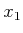,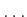,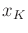,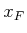generated by a trial. Let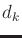denote a temporal difference, which is defined as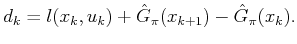(10.86)

Note that both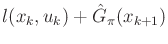and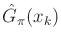could each serve as an estimate of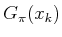. The difference is that the right part of (10.87) utilizes the latest cost obtained from the simulator for the first step and then uses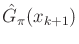for an estimate of the remaining cost. In this and subsequent expressions, every action,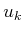, is chosen using the plan: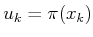.

Let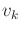denote the number of times that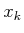has been visited so far, for each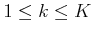, including previous trials and the current visit. The following update algorithm can be used during the trial. When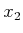is reached, the value atis updated as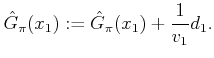(10.87)

When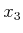is reached, the values atandare updated as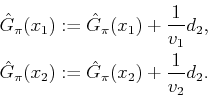(10.88)

Now consider what has been done so far at. The temporal differences partly collapse: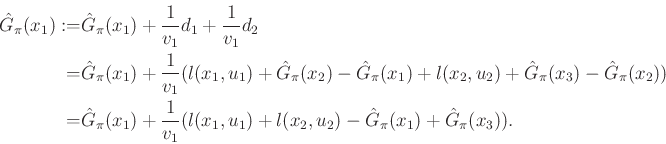(10.89)

When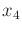is reached, similar updates are performed. At, the updates are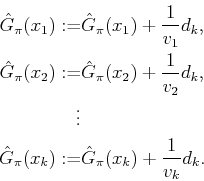(10.90)

The updates are performed in this way until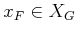is reached. Now consider what was actually computed for each. The temporal differences form a telescoping sum that collapses, as shown in (10.90) after two iterations. After all iterations have been completed, the value athas been updated as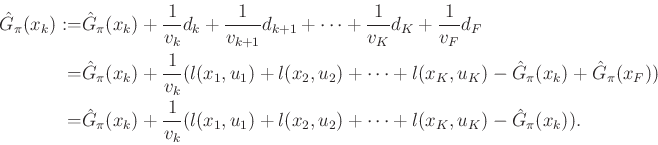(10.91)

The final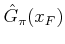was deleted because its value is zero, assuming that the termination action is applied by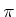. The resulting final expression is equivalent to (10.85) if each visited state in the sequence was distinct. This is often not true, which makes the method discussed above differ slightly from the method of (10.85) because the count,, may change during the trial in the temporal difference scheme. This difference, however, is negligible, and the temporal difference method computes estimates that converge to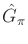[96,97].

The temporal difference method presented so far can be generalized in a way that often leads to faster convergence in practice. Let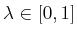be a specified parameter. The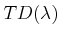temporal difference method replaces the equations in (10.91) with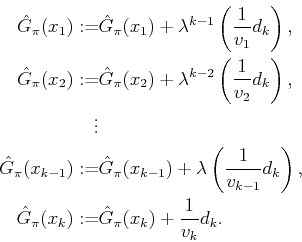(10.92)

This has the effect of discounting costs that are received far away from. The method in (10.91) was the special case of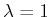, yielding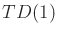.

Another interesting special case is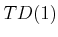, which becomes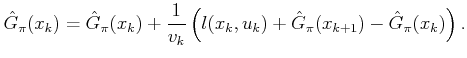(10.93)

This form appears most often in reinforcement learning literature (although it is applied to the discounted-cost model instead). Experimental evidence indicates that lower values of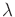help to improve the convergence rate. Convergence for all values ofis proved in .

One source of intuition about why (10.94) works is that it is a special case of a stochastic iterative algorithm or the Robbins-Monro algorithm [88,97,566]. This is a general statistical estimation technique that is used for solving systems of the form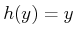by using a sequence of samples. Each sample represents a measurement of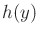using Monte Carlo simulation. The general form of this iterative approach is to update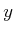as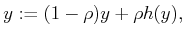(10.94)

in which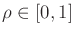is a parameter whose choice affects the convergence rate. Intuitively, (10.95) updatesby interpolating between its original value and the most recent sample of. Convergence proofs for this algorithm are not given here; see  for details. The typical behavior is that a smaller value of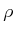leads to more reliable estimates when there is substantial noise in the simulation process, but this comes at the cost of slowing the convergence rate. The convergence is asymptotic, which requires that all edges (that have nonzero probability) in the plan-based state transition graph should be visited infinitely often.

A general approach to obtainingcan be derived within the stochastic iterative framework by generalizing: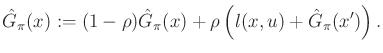(10.95)

The formulation ofin (10.94) essentially selects theparameter by the way it was derived, but in (10.96) any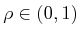may be used.

It may appear incorrect that the update equation does not take into account the transition probabilities. It turns out that they are taken into account in the simulation process because transitions that are more likely to occur have a stronger effect on (10.96). The same thing occurs when the mean of a nonuniform probability density function is estimated by using samples from the distribution. The values that occur with higher frequency make stronger contributions to the average, which automatically gives them the appropriate weight.

Steven M LaValle 2020-08-14